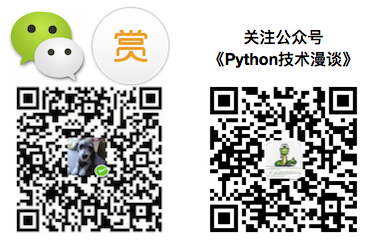# 1   摘要

https://github.com/zhengwh/captcha-svm

# 4   引言

http://www.cnblogs.com/beer/p/4996833.html

• python3.5

python SDK版本

• PIL

图片处理库

• libsvm

开源的svm机器学习库

# 6   基本流程

1. 准备原始图片素材
2. 图片预处理
3. 图片字符切割
4. 图片尺寸归一化
5. 图片字符标记
6. 字符图片特征提取
7. 生成特征和标记对应的训练数据集
8. 训练特征标记数据生成识别模型
9. 使用识别模型预测新的未知图片集
10. 达到根据“图片”就能返回识别正确的字符集的目标

# 7   素材准备

## 7.1   素材选择1. 由纯阿拉伯数字组成
2. 字数为4位
3. 字符排列有规律
4. 字体是用的统一字体

1. 图片背景有干扰噪点

## 7.2   素材获取

1. 通过浏览器的抓包功能获取随机图片验证码生成接口
2. 批量请求接口以获取图片
3. 将图片保存到本地磁盘目录中

def downloads_pic(**kwargs):
pic_name = kwargs.get('pic_name', None)

res = requests.get(url, stream=True)
with open(pic_path + pic_name+'.bmp', 'wb') as f:
for chunk in res.iter_content(chunk_size=1024):
if chunk:  # filter out keep-alive new chunks
f.write(chunk)
f.flush()
f.close()# 8   图片预处理

1. 读取原始图片素材
2. 将彩色图片二值化为黑白图片
3. 去除背景噪点

## 8.1   二值化图片

1. 将RGB彩图转为灰度图
2. 将灰度图按照设定阈值转化为二值图
image = Image.open(img_path)
imgry = image.convert('L')  # 转化为灰度图

table = get_bin_table()
out = imgry.point(table, '1')

def get_bin_table(threshold=140):
"""
获取灰度转二值的映射table
:param threshold:
:return:
"""
table = []
for i in range(256):
if i < threshold:
table.append(0)
else:
table.append(1)

return table

1111000111111000111111100001111100000011
1110111011110111011111011110111100110111
1001110011110111101011011010101101110111
1101111111110110101111110101111111101111
1101000111110111001111110011111111101111
1100111011111000001111111001011111011111
1101110001111111101011010110111111011111
1101111011111111101111011110111111011111
1101111011110111001111011110111111011100
1110000111111000011101100001110111011111

## 8.2   去除噪点

• 对某个 黑点 周边的九宫格里面的黑色点计数
• 如果黑色点少于2个则证明此点为孤立点，然后得到所有的孤立点
• 对所有孤立点一次批量移除。

• 顶点A
• 非顶点的边界点B
• 内部点C• A类点计算周边相邻的3个点（如上图红框所示）
• B类点计算周边相邻的5个点（如上图红框所示）
• C类点计算周边相邻的8个点（如上图红框所示）

• A类点继续细分为：左上，左下，右上，右下
• B类点继续细分为：上，下，左，右
• C类点不用细分

def sum_9_region(img, x, y):
"""
9邻域框,以当前点为中心的田字框,黑点个数
:param x:
:param y:
:return:
"""
# todo 判断图片的长宽度下限
cur_pixel = img.getpixel((x, y))  # 当前像素点的值
width = img.width
height = img.height

if cur_pixel == 1:  # 如果当前点为白色区域,则不统计邻域值
return 0

if y == 0:  # 第一行
if x == 0:  # 左上顶点,4邻域
# 中心点旁边3个点
sum = cur_pixel \
+ img.getpixel((x, y + 1)) \
+ img.getpixel((x + 1, y)) \
+ img.getpixel((x + 1, y + 1))
return 4 - sum
elif x == width - 1:  # 右上顶点
sum = cur_pixel \
+ img.getpixel((x, y + 1)) \
+ img.getpixel((x - 1, y)) \
+ img.getpixel((x - 1, y + 1))

return 4 - sum
else:  # 最上非顶点,6邻域
sum = img.getpixel((x - 1, y)) \
+ img.getpixel((x - 1, y + 1)) \
+ cur_pixel \
+ img.getpixel((x, y + 1)) \
+ img.getpixel((x + 1, y)) \
+ img.getpixel((x + 1, y + 1))
return 6 - sum
elif y == height - 1:  # 最下面一行
if x == 0:  # 左下顶点
# 中心点旁边3个点
sum = cur_pixel \
+ img.getpixel((x + 1, y)) \
+ img.getpixel((x + 1, y - 1)) \
+ img.getpixel((x, y - 1))
return 4 - sum
elif x == width - 1:  # 右下顶点
sum = cur_pixel \
+ img.getpixel((x, y - 1)) \
+ img.getpixel((x - 1, y)) \
+ img.getpixel((x - 1, y - 1))

return 4 - sum
else:  # 最下非顶点,6邻域
sum = cur_pixel \
+ img.getpixel((x - 1, y)) \
+ img.getpixel((x + 1, y)) \
+ img.getpixel((x, y - 1)) \
+ img.getpixel((x - 1, y - 1)) \
+ img.getpixel((x + 1, y - 1))
return 6 - sum
else:  # y不在边界
if x == 0:  # 左边非顶点
sum = img.getpixel((x, y - 1)) \
+ cur_pixel \
+ img.getpixel((x, y + 1)) \
+ img.getpixel((x + 1, y - 1)) \
+ img.getpixel((x + 1, y)) \
+ img.getpixel((x + 1, y + 1))

return 6 - sum
elif x == width - 1:  # 右边非顶点
# print('%s,%s' % (x, y))
sum = img.getpixel((x, y - 1)) \
+ cur_pixel \
+ img.getpixel((x, y + 1)) \
+ img.getpixel((x - 1, y - 1)) \
+ img.getpixel((x - 1, y)) \
+ img.getpixel((x - 1, y + 1))

return 6 - sum
else:  # 具备9领域条件的
sum = img.getpixel((x - 1, y - 1)) \
+ img.getpixel((x - 1, y)) \
+ img.getpixel((x - 1, y + 1)) \
+ img.getpixel((x, y - 1)) \
+ cur_pixel \
+ img.getpixel((x, y + 1)) \
+ img.getpixel((x + 1, y - 1)) \
+ img.getpixel((x + 1, y)) \
+ img.getpixel((x + 1, y + 1))
return 9 - sum

Tips:这个地方是相当考验人的细心和耐心程度了，这个地方的工作量还是蛮大的，花了半个晚上的时间才完成的。# 9   图片字符切割

## 9.1   分割算法• 整个图片尺寸是 40*10
• 单个字符尺寸是 6*10
• 左右字符和左右边缘相距2个像素
• 字符上下紧挨边缘（即相距0个像素）

def get_crop_imgs(img):
"""
按照图片的特点,进行切割,这个要根据具体的验证码来进行工作. # 见原理图
:param img:
:return:
"""
child_img_list = []
for i in range(4):
x = 2 + i * (6 + 4)  # 见原理图
y = 0
child_img = img.crop((x, y, x + 6, y + 10))
child_img_list.append(child_img)

return child_img_list## 9.2   内容小结

• 4位字符和40000位字符的验证码区别不大

• 纯数字 和 数字及字母组合 的验证码区别不大
• 纯数字。分类数为10

• 纯字母
• 不区分大小写。分类数为26
• 区分大小写。分类数为52
• 数字和区分大小写的字母组合。分类数为62

# 11   模型训练步骤

1. 大量完成预处理并切割到原子级的图片素材准备
2. 对素材图片进行人为分类，即：打标签
3. 定义单张图片的识别特征
4. 使用SVM训练模型对打了标签的特征文件进行训练，得到模型文件

# 12   素材准备# 13   素材标记

1. 为0~9每个数字建立一个目录，目录名称为相应数字（相当于标签）

2. 人为判定 图片内容，并将图片拖到指定数字目录中

3. 每个目录中存放100张左右的素材

一般情况下，标记的素材越多，那么训练出的模型的分辨能力和预测能力越强。例如本文中，标记素材为十多张的时候，对新的测试图片识别率基本为零，但是到达100张时，则可以达到近乎100%的识别率# 14   特征选择1. 每行上黑色像素的个数，可以得到10个特征
2. 每列上黑色像素的个数，可以得到6个特征

def get_feature(img):
"""
获取指定图片的特征值,
1. 按照每排的像素点,高度为10,则有10个维度,然后为6列,总共16个维度
:param img_path:
:return:一个维度为10（高度）的列表
"""

width, height = img.size

pixel_cnt_list = []
height = 10
for y in range(height):
pix_cnt_x = 0
for x in range(width):
if img.getpixel((x, y)) == 0:  # 黑色点
pix_cnt_x += 1

pixel_cnt_list.append(pix_cnt_x)

for x in range(width):
pix_cnt_y = 0
for y in range(height):
if img.getpixel((x, y)) == 0:  # 黑色点
pix_cnt_y += 1

pixel_cnt_list.append(pix_cnt_y)

return pixel_cnt_list1. 第一列是标签列，即此图片人为标记值，后续还有其它数值1~9的标记
2. 后面是16组特征值，冒号前面是索引号，后面是值
3. 如果有1000张训练图片，那么会产生1000行的记录

# 15   模型训练

def train_svm_model():
"""
训练并生成model文件
:return:
"""
y, x = svm_read_problem(svm_root + '/train_pix_feature_xy.txt')
model = svm_train(y, x)
svm_save_model(model_path, model)

# 16   模型测试

• 使用一组全部标记为8的21张图片来进行模型测试
• 测试图片生成带标记的特征文件名称为 last_test_pix_xy_new.txt

1. 到60张左右的时候，正确率大概80%
2. 到185张的时候，正确率基本上达到100%

def svm_model_test():
"""
使用测试集测试模型
:return:
"""
yt, xt = svm_read_problem(svm_root + '/last_test_pix_xy_new.txt')
p_label, p_acc, p_val = svm_predict(yt, xt, model)#p_label即为识别的结果

cnt = 0
for item in p_label:
print('%d' % item, end=',')
cnt += 1
if cnt % 8 == 0:
print('')

# 17   完整识别流程

1. 传入一组验证码图片
2. 对图片进行预处理：去噪，二值等等
3. 切割成4张有序的单字符图片
4. 使用模型文件分别对4张图片进行识别
5. 将识别结果拼接
6. 返回识别结果# 18   效率优化

1. 将图片资源的网络请求部分做成异步非阻塞模式
2. 利用好多核CPU，多进程并行运行
3. 在图片特征上认真挑选和实验，降低维度

1. 粗暴地增加CPU性能
2. 粗暴地增加运行机器

# 19   互联网安全警示

• 12306火车售票网，春节期间早上8:00某车次放出的500张票，1s内全部被抢光，最后发现正常需求的人抢不到票，但是黄牛却大大的有票
• 某某手机网站，早上10:00开启抢购活动，守候了许久的无数的你都铩羽而归，但是同样黄牛却大量有货

Web应用系统的小安全漏洞及相应的攻击方式

http://www.cnblogs.com/beer/p/4814587.html

1. 目前确实有一些web应用系统连验证码都没有，只能任人宰割
2. 即使web应用系统有验证码但是难度不够，也只能任人宰割

# 20   积极应用场景

• 银行卡号识别
• 身份证号识别
• 车牌号码识别

1. 字体单一
2. 字符为简单的数字或字母组合
3. 文字的排列是标准化统一化的

# 21   小结

1. 对于掌握OCR技术的人
• 不要做违法的事，因为目前被抓的“白帽子”的新闻也蛮多的
• 在不违法的情况下，还是可以向存在漏洞的系统管理员提出善意提醒
• 以自己的专业知识，多做一些促进社会进步，提升社会生产力的事情，如纸书电子化等等
2. 对于仍然沿用旧的落后的IT系统的公司或者机构相关人员

应该尽快认识到事情的严重性，赶紧升级自己的系统，或者将这一块业务交付给专门的安全公司

# 22   参考资料

  LibSVM for Python 使用 http://www.cnblogs.com/Finley/p/5329417.html
  基于SVM的手写体阿拉伯数字识别.张鸽,陈书开.长沙理工大学计算机通讯工程学院.2005

# 如果您觉得本文确实帮助了您，可以微信扫一扫，进行小额的打赏和鼓励，谢谢 ^_^ 或者关注我的微信公众号，更多技术文章等你来发现，posted @ 2016-07-15 12:03  一点一滴的Beer  阅读(167546)  评论(56编辑  收藏  举报